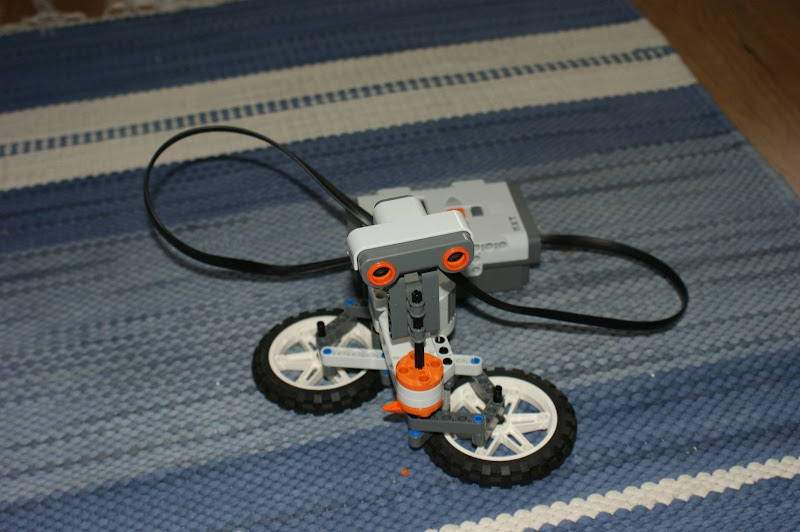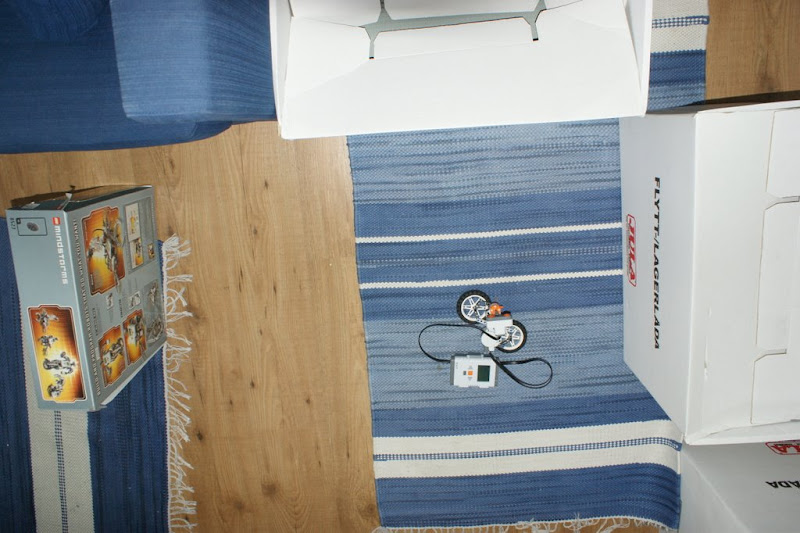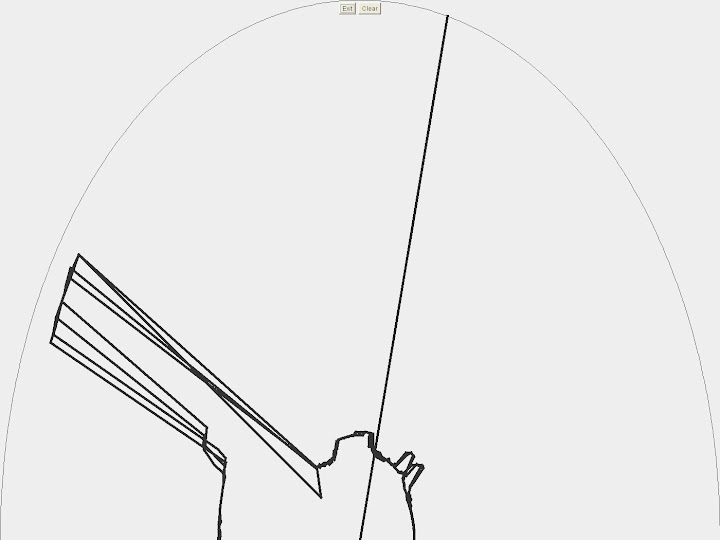## 2009-06-21

### Going the Right Way

Now we've finally begun to build something that with a bit of imagination remotely resembles our ultimate goal. UltrasonicScanner is a stationary robot which is much like the UltrasonicTest, but now we've integrated communication between the computer and the NXT through Bluetooth and we can let the computer do all the calculation and show the results graphically.

UltrasonicScaner
http://code.google.com/p/penemunxt/source/browse/#svn/trunk/UltrasonicScannerClient/src
http://code.google.com/p/penemunxt/source/browse/#svn/trunk/UltrasonicScannerServer/srcThe code is more or less CommunicationTest combined with UltrasonicTest. We've established streaming of both the ultrasonic sensor getDistance() and motor getTachoCount() (which returns the angle from it's original position) and then perform all the necessary calculations serverside. We also have a third "channel" streaming data to allow us to give commands in both directions. This means that both the NXT and the JAVA application have the ability to close both programs.

` private Point getScreenPos(int Distance, int Angle) {Angle += 90;int x, y;int distx, disty;distx = (int) (((Math.min(ULTRASONIC_SENSOR_MAX_DISTANCE, Distance) / (double) ULTRASONIC_SENSOR_MAX_DISTANCE) * (getWidth() / 2)));disty = (int) ((Math.min(ULTRASONIC_SENSOR_MAX_DISTANCE, Distance) / (double) ULTRASONIC_SENSOR_MAX_DISTANCE) * getHeight());x = (int) ((distx * Math.cos((Angle) * Math.PI / 180)) + (getWidth() / 2));x = getWidth() + (x * -1);y = (int) (-1 * (disty * Math.sin(Angle * Math.PI / 180)) + getHeight());return new Point(x, y);}`

This is the algorithm we use to calculate the coordinates on the screen based on the data from the NXT, and it's all straightforward mathematics.As you can see on these pictures the "map" the robot managed to create isn't totally accurate. Apparently the sensor can't give accurate data when facing at an angle to a flat surface, with the result that flat surfaces seems to be curved around the robot. Once we have the robot mobile a lot of this problem should be solved.

If check out our videos you can also see a version before we implemented Bluetooth for it.

This is the final result:

#### Skicka en kommentar